# RD Sharma Solutions for Class 8 Maths Chapter 1 - Rational Numbers Exercise 1.6

Students can refer and download RD Sharma Solutions for Class 8 Maths Exercise 1.6 Chapter 1, Rational Numbers from the links provided below.

Our experts have solved the RD Sharma Solutions to ensure that the students are thorough with their basic concepts and help clear their doubts. Exercise 1.6 is based on the properties of rational numbers which includes the existence of a multiplicative identity, distributivity of multiplication over addition, multiplication by 0. Students can download the RD Sharma Class 8 Solutions Maths Chapter 1 and practise them offline.

## Download PDF of RD Sharma Solutions for Class 8 Maths Exercise 1.6 Chapter 1 Rational Numbers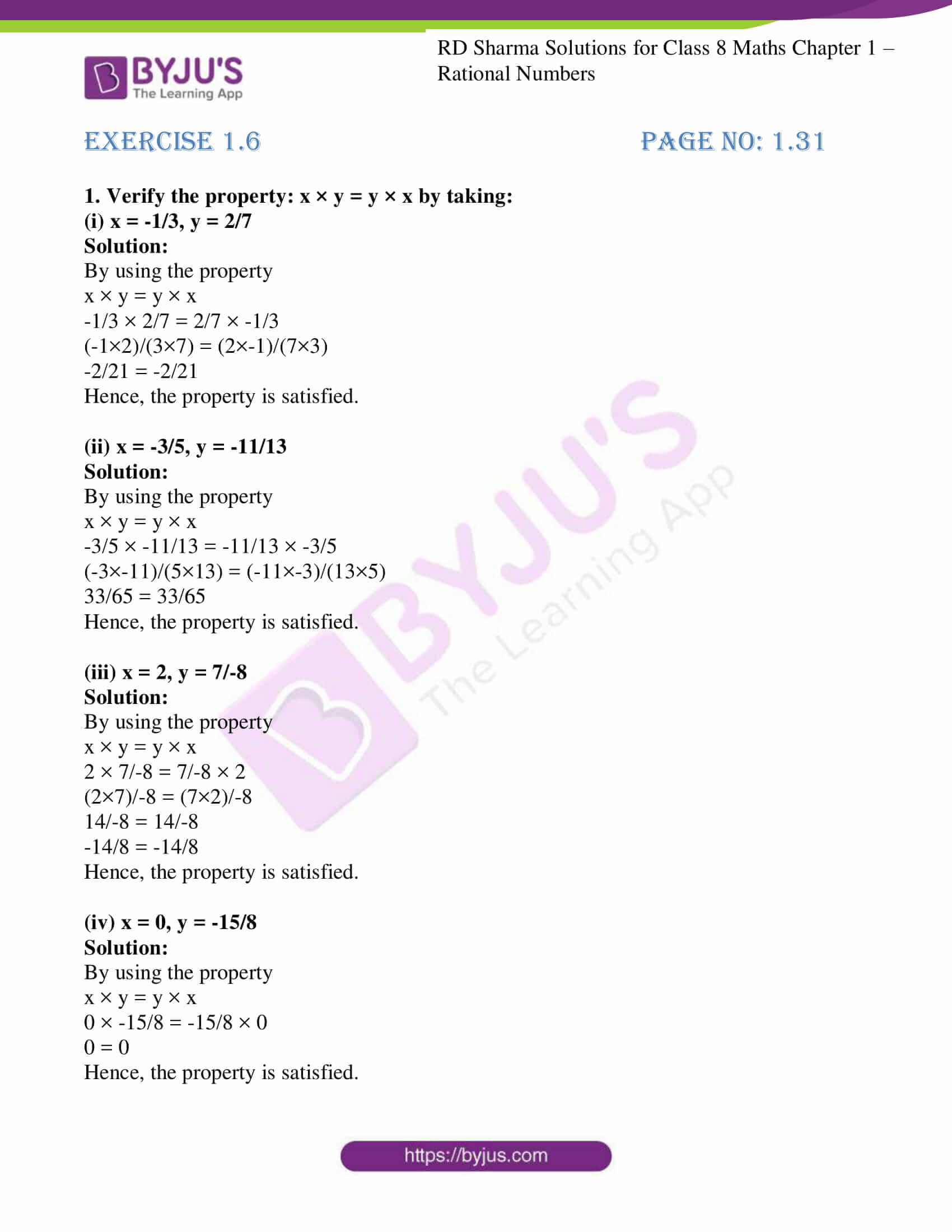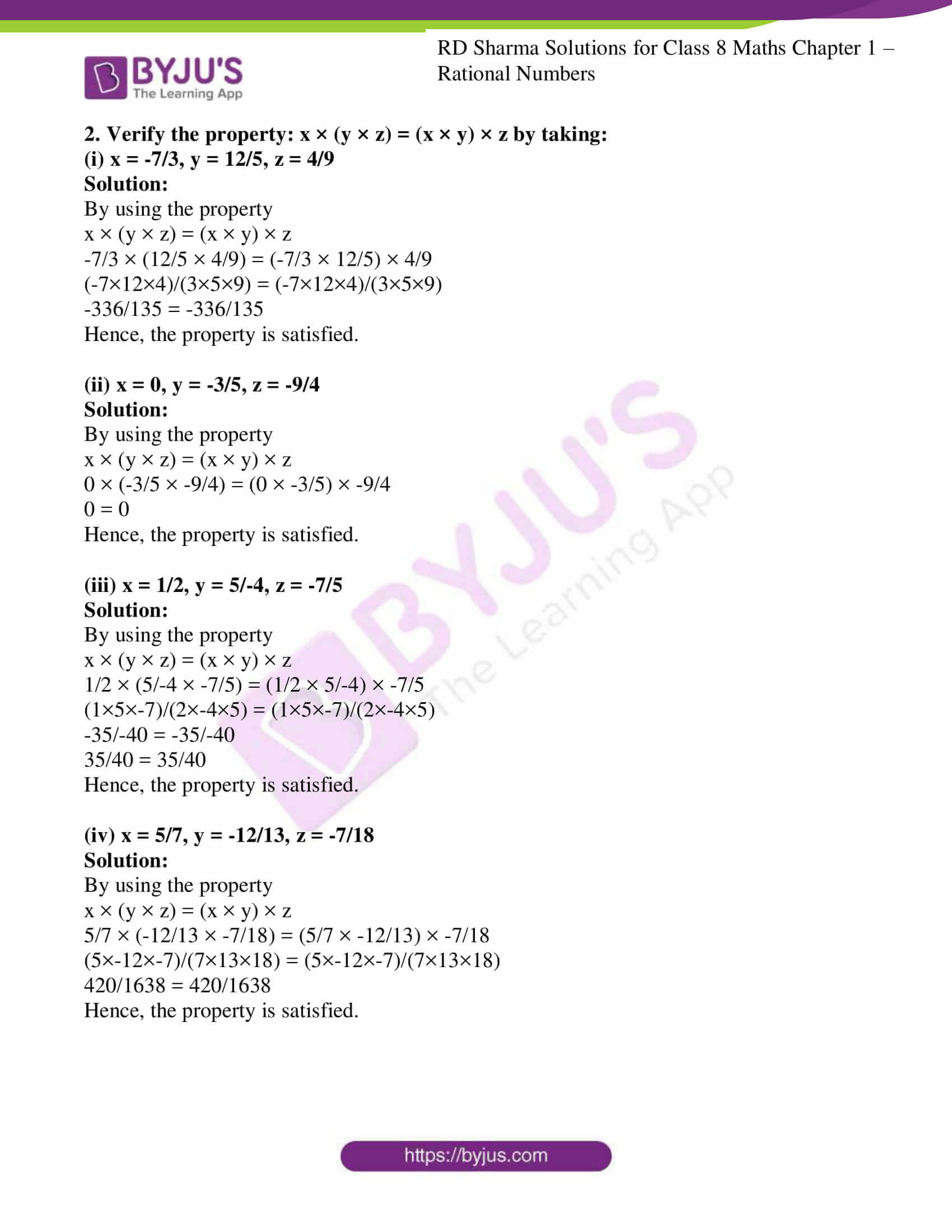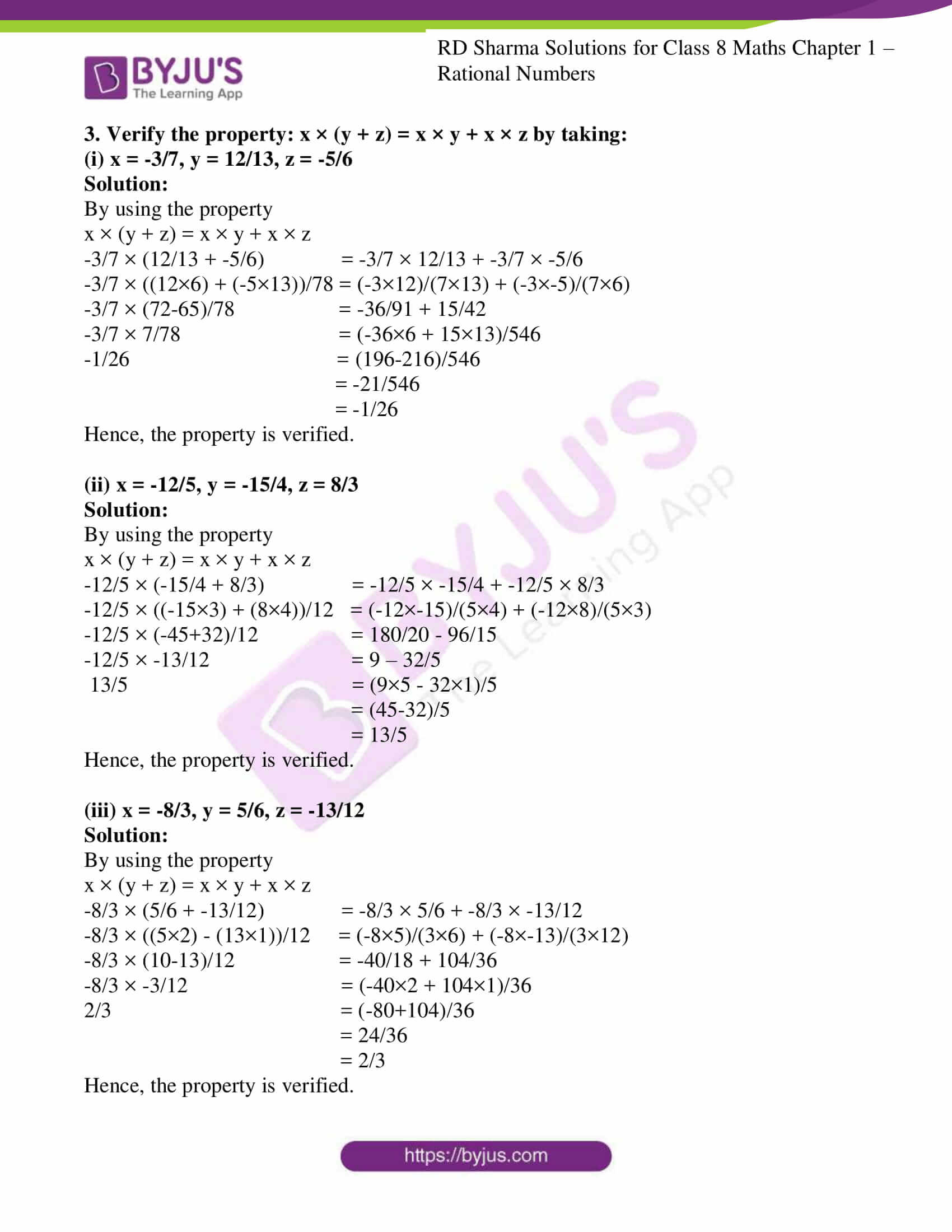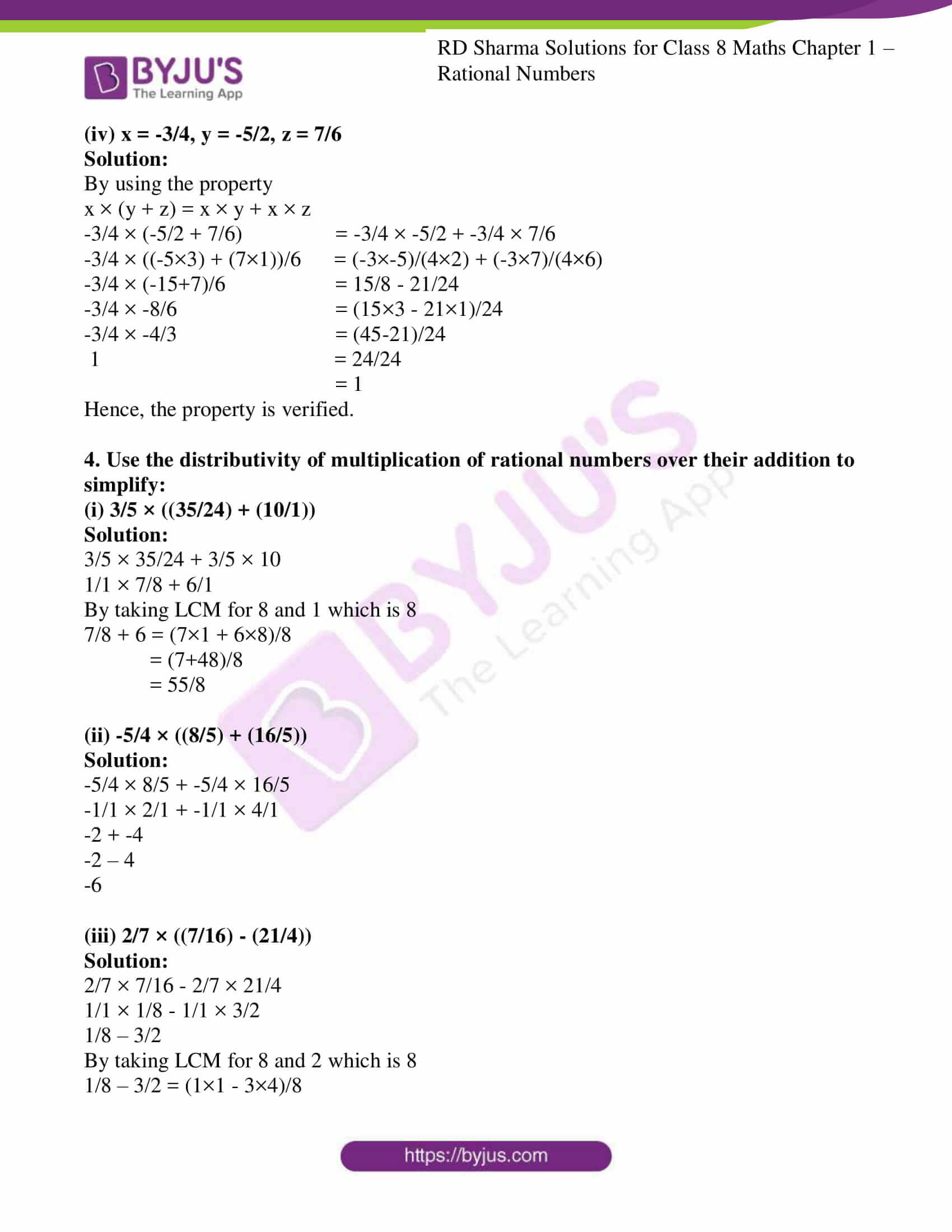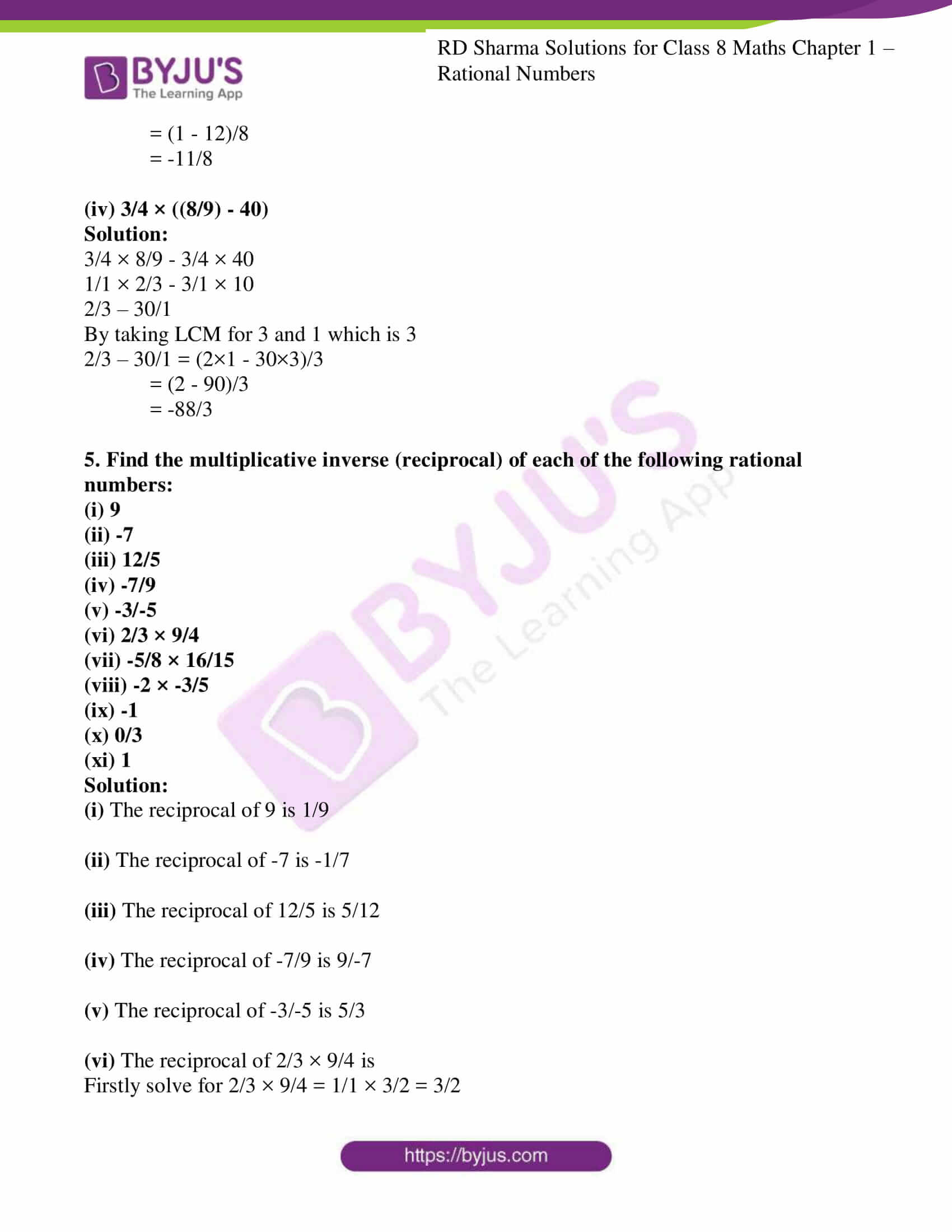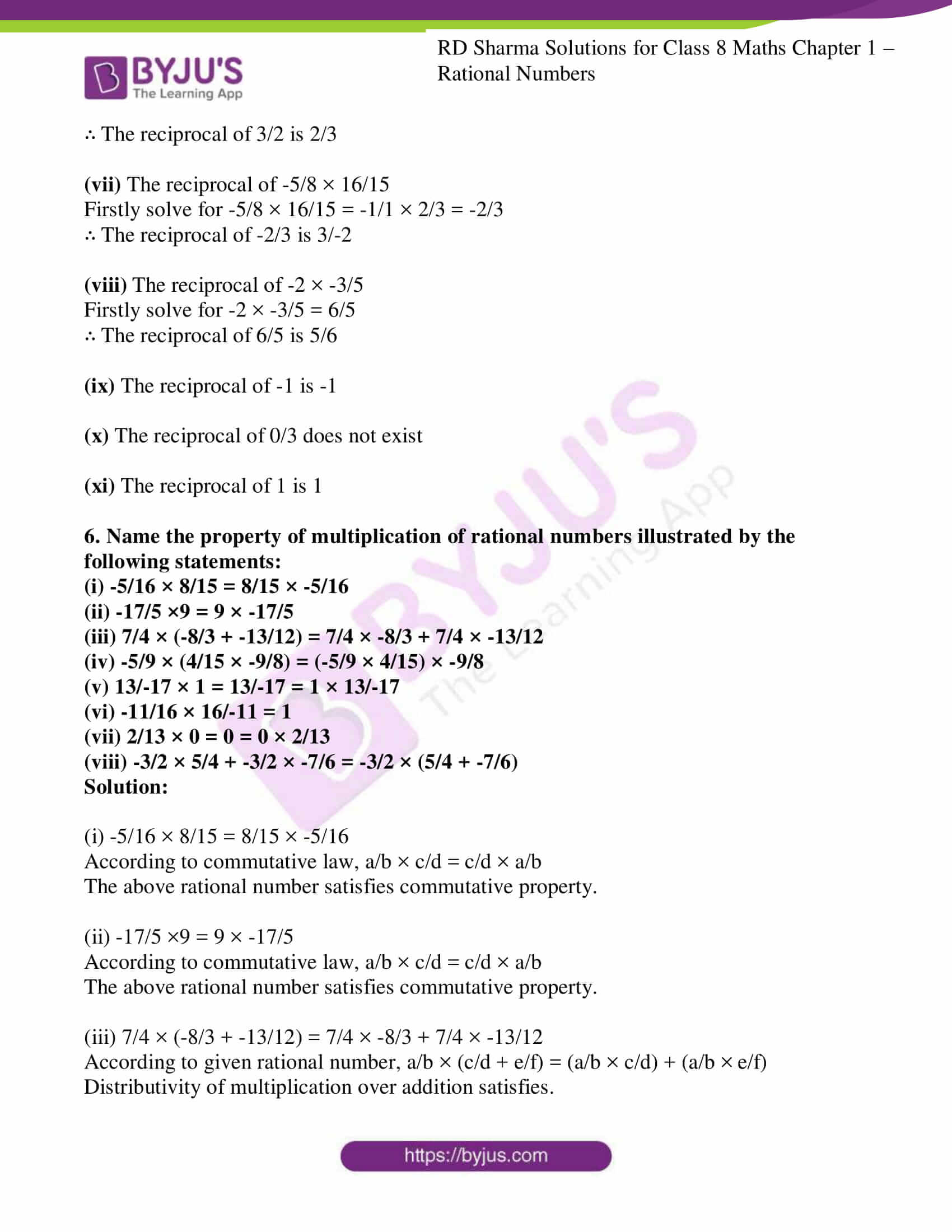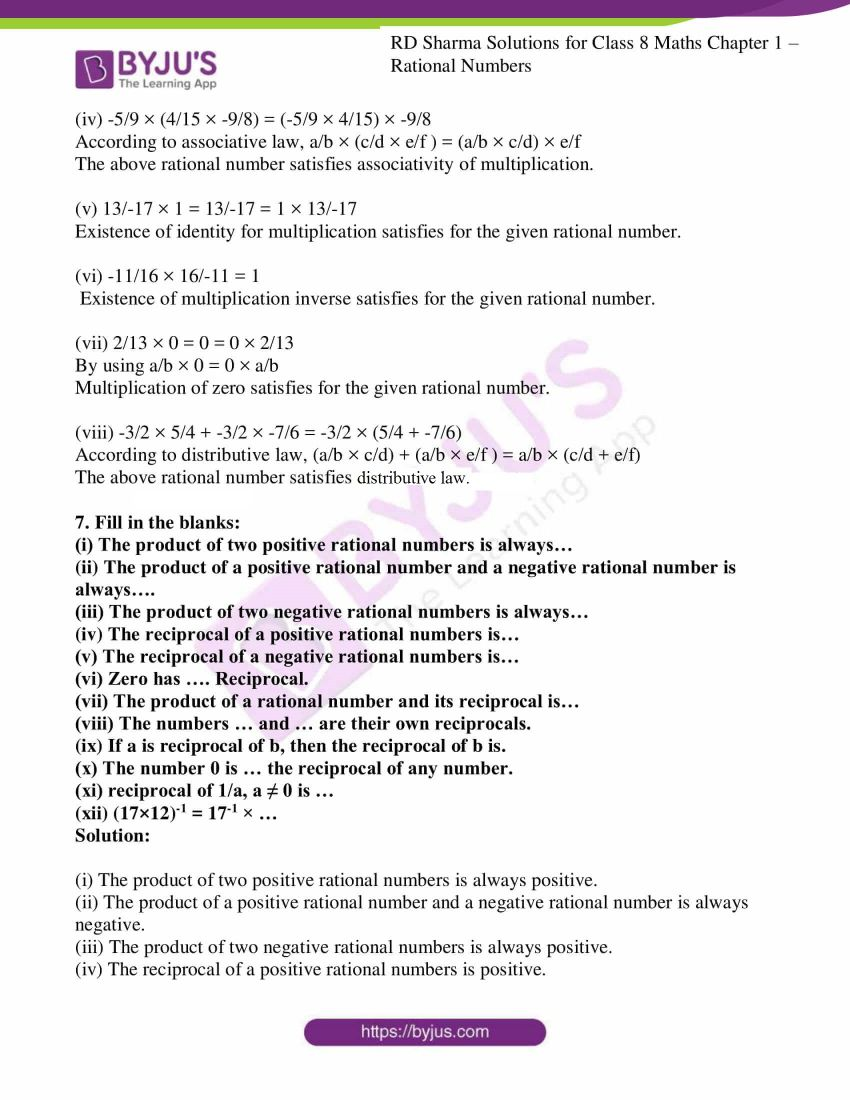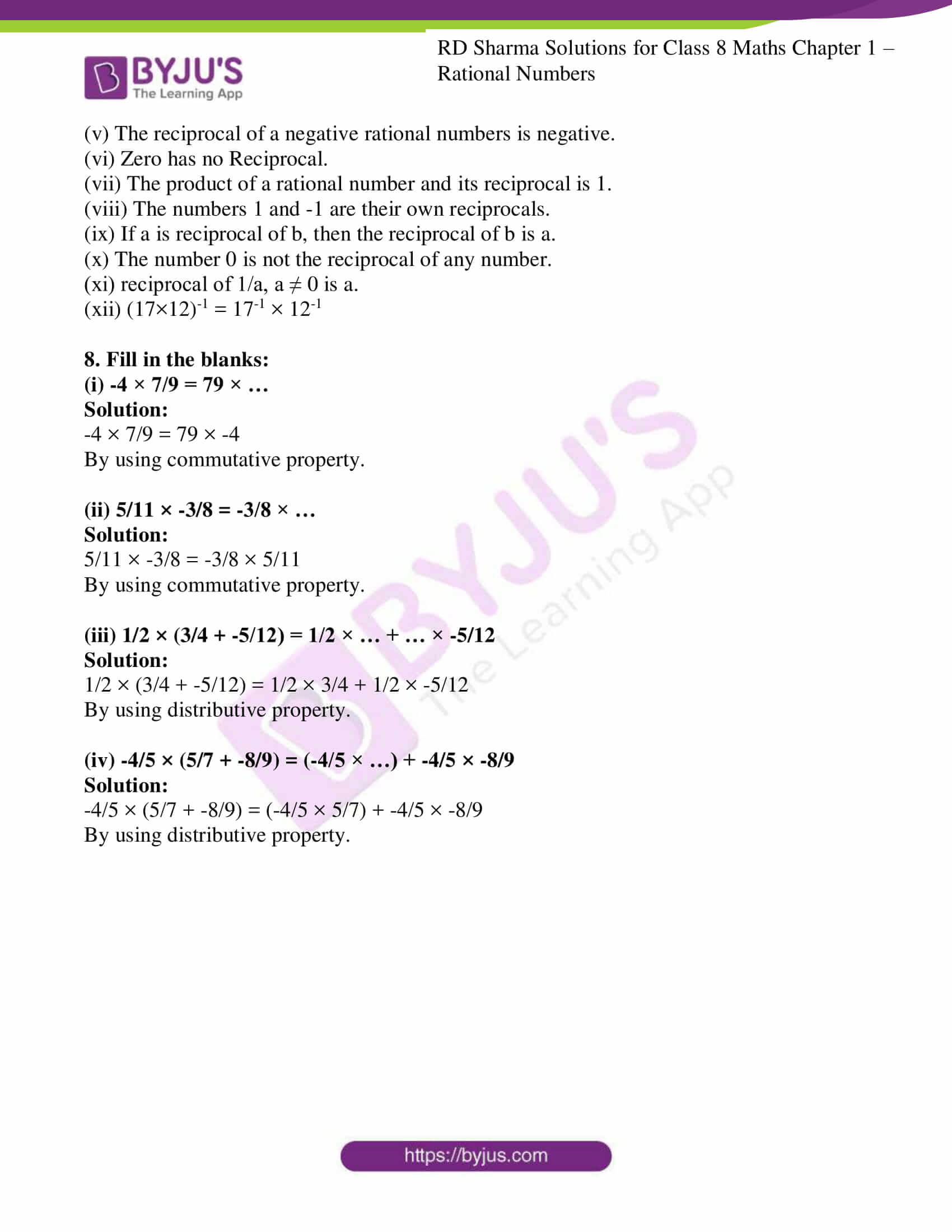### Access Answers to RD Sharma Solutions for Class 8 Maths Exercise 1.6 Chapter 1 Rational Numbers

1. Verify the property: x × y = y × x by taking:

(i) x = -1/3, y = 2/7

Solution:

By using the property

x × y = y × x

-1/3 × 2/7 = 2/7 × -1/3

(-1×2)/(3×7) = (2×-1)/(7×3)

-2/21 = -2/21

Hence, the property is satisfied.

(ii) x = -3/5, y = -11/13

Solution:

By using the property

x × y = y × x

-3/5 × -11/13 = -11/13 × -3/5

(-3×-11)/(5×13) = (-11×-3)/(13×5)

33/65 = 33/65

Hence, the property is satisfied.

(iii) x = 2, y = 7/-8

Solution:

By using the property

x × y = y × x

2 × 7/-8 = 7/-8 × 2

(2×7)/-8 = (7×2)/-8

14/-8 = 14/-8

-14/8 = -14/8

Hence, the property is satisfied.

(iv) x = 0, y = -15/8

Solution:

By using the property

x × y = y × x

0 × -15/8 = -15/8 × 0

0 = 0

Hence, the property is satisfied.

2. Verify the property: x × (y × z) = (x × y) × z by taking:

(i) x = -7/3, y = 12/5, z = 4/9

Solution:

By using the property

x × (y × z) = (x × y) × z

-7/3 × (12/5 × 4/9) = (-7/3 × 12/5) × 4/9

(-7×12×4)/(3×5×9) = (-7×12×4)/(3×5×9)

-336/135 = -336/135

Hence, the property is satisfied.

(ii) x = 0, y = -3/5, z = -9/4

Solution:

By using the property

x × (y × z) = (x × y) × z

0 × (-3/5 × -9/4) = (0 × -3/5) × -9/4

0 = 0

Hence, the property is satisfied.

(iii) x = 1/2, y = 5/-4, z = -7/5

Solution:

By using the property

x × (y × z) = (x × y) × z

1/2 × (5/-4 × -7/5) = (1/2 × 5/-4) × -7/5

(1×5×-7)/(2×-4×5) = (1×5×-7)/(2×-4×5)

-35/-40 = -35/-40

35/40 = 35/40

Hence, the property is satisfied.

(iv) x = 5/7, y = -12/13, z = -7/18

Solution:

By using the property

x × (y × z) = (x × y) × z

5/7 × (-12/13 × -7/18) = (5/7 × -12/13) × -7/18

(5×-12×-7)/(7×13×18) = (5×-12×-7)/(7×13×18)

420/1638 = 420/1638

Hence, the property is satisfied.

3. Verify the property: x × (y + z) = x × y + x × z by taking:

(i) x = -3/7, y = 12/13, z = -5/6

Solution:

By using the property

x × (y + z) = x × y + x × z

-3/7 × (12/13 + -5/6) = -3/7 × 12/13 + -3/7 × -5/6

-3/7 × ((12×6) + (-5×13))/78 = (-3×12)/(7×13) + (-3×-5)/(7×6)

-3/7 × (72-65)/78 = -36/91 + 15/42

-3/7 × 7/78 = (-36×6 + 15×13)/546

-1/26 = (196-216)/546

= -21/546

= -1/26

Hence, the property is verified.

(ii) x = -12/5, y = -15/4, z = 8/3

Solution:

By using the property

x × (y + z) = x × y + x × z

-12/5 × (-15/4 + 8/3) = -12/5 × -15/4 + -12/5 × 8/3

-12/5 × ((-15×3) + (8×4))/12 = (-12×-15)/(5×4) + (-12×8)/(5×3)

-12/5 × (-45+32)/12 = 180/20 – 96/15

-12/5 × -13/12 = 9 – 32/5

13/5 = (9×5 – 32×1)/5

= (45-32)/5

= 13/5

Hence, the property is verified.

(iii) x = -8/3, y = 5/6, z = -13/12

Solution:

By using the property

x × (y + z) = x × y + x × z

-8/3 × (5/6 + -13/12) = -8/3 × 5/6 + -8/3 × -13/12

-8/3 × ((5×2) – (13×1))/12 = (-8×5)/(3×6) + (-8×-13)/(3×12)

-8/3 × (10-13)/12 = -40/18 + 104/36

-8/3 × -3/12 = (-40×2 + 104×1)/36

2/3 = (-80+104)/36

= 24/36

= 2/3

Hence, the property is verified.

(iv) x = -3/4, y = -5/2, z = 7/6

Solution:

By using the property

x × (y + z) = x × y + x × z

-3/4 × (-5/2 + 7/6) = -3/4 × -5/2 + -3/4 × 7/6

-3/4 × ((-5×3) + (7×1))/6 = (-3×-5)/(4×2) + (-3×7)/(4×6)

-3/4 × (-15+7)/6 = 15/8 – 21/24

-3/4 × -8/6 = (15×3 – 21×1)/24

-3/4 × -4/3 = (45-21)/24

1 = 24/24

= 1

Hence, the property is verified.

4. Use the distributivity of multiplication of rational numbers over their addition to simplify:

(i) 3/5 × ((35/24) + (10/1))

Solution:

3/5 × 35/24 + 3/5 × 10

1/1 × 7/8 + 6/1

By taking LCM for 8 and 1 which is 8

7/8 + 6 = (7×1 + 6×8)/8

= (7+48)/8

= 55/8

(ii) -5/4 × ((8/5) + (16/5))

Solution:

-5/4 × 8/5 + -5/4 × 16/5

-1/1 × 2/1 + -1/1 × 4/1

-2 + -4

-2 – 4

-6

(iii) 2/7 × ((7/16) – (21/4))

Solution:

2/7 × 7/16 – 2/7 × 21/4

1/1 × 1/8 – 1/1 × 3/2

1/8 – 3/2

By taking LCM for 8 and 2 which is 8

1/8 – 3/2 = (1×1 – 3×4)/8

= (1 – 12)/8

= -11/8

(iv) 3/4 × ((8/9) – 40)

Solution:

3/4 × 8/9 – 3/4 × 40

1/1 × 2/3 – 3/1 × 10

2/3 – 30/1

By taking LCM for 3 and 1 which is 3

2/3 – 30/1 = (2×1 – 30×3)/3

= (2 – 90)/3

= -88/3

5. Find the multiplicative inverse (reciprocal) of each of the following rational numbers:

(i) 9

(ii) -7

(iii) 12/5

(iv) -7/9

(v) -3/-5

(vi) 2/3 × 9/4

(vii) -5/8 × 16/15

(viii) -2 × -3/5

(ix) -1

(x) 0/3

(xi) 1

Solution:

(i) The reciprocal of 9 is 1/9

(ii) The reciprocal of -7 is -1/7

(iii) The reciprocal of 12/5 is 5/12

(iv) The reciprocal of -7/9 is 9/-7

(v) The reciprocal of -3/-5 is 5/3

(vi) The reciprocal of 2/3 × 9/4 is

Firstly solve for 2/3 × 9/4 = 1/1 × 3/2 = 3/2

∴ The reciprocal of 3/2 is 2/3

(vii) The reciprocal of -5/8 × 16/15

Firstly solve for -5/8 × 16/15 = -1/1 × 2/3 = -2/3

∴ The reciprocal of -2/3 is 3/-2

(viii) The reciprocal of -2 × -3/5

Firstly solve for -2 × -3/5 = 6/5

∴ The reciprocal of 6/5 is 5/6

(ix) The reciprocal of -1 is -1

(x) The reciprocal of 0/3 does not exist

(xi) The reciprocal of 1 is 1

6. Name the property of multiplication of rational numbers illustrated by the following statements:

(i) -5/16 × 8/15 = 8/15 × -5/16

(ii) -17/5 ×9 = 9 × -17/5

(iii) 7/4 × (-8/3 + -13/12) = 7/4 × -8/3 + 7/4 × -13/12

(iv) -5/9 × (4/15 × -9/8) = (-5/9 × 4/15) × -9/8

(v) 13/-17 × 1 = 13/-17 = 1 × 13/-17

(vi) -11/16 × 16/-11 = 1

(vii) 2/13 × 0 = 0 = 0 × 2/13

(viii) -3/2 × 5/4 + -3/2 × -7/6 = -3/2 × (5/4 + -7/6)

Solution:

(i) -5/16 × 8/15 = 8/15 × -5/16

According to commutative law, a/b × c/d = c/d × a/b

The above rational number satisfies commutative property.

(ii) -17/5 ×9 = 9 × -17/5

According to commutative law, a/b × c/d = c/d × a/b

The above rational number satisfies commutative property.

(iii) 7/4 × (-8/3 + -13/12) = 7/4 × -8/3 + 7/4 × -13/12

According to given rational number, a/b × (c/d + e/f) = (a/b × c/d) + (a/b × e/f)

Distributivity of multiplication over addition satisfies.

(iv) -5/9 × (4/15 × -9/8) = (-5/9 × 4/15) × -9/8

According to associative law, a/b × (c/d × e/f ) = (a/b × c/d) × e/f

The above rational number satisfies associativity of multiplication.

(v) 13/-17 × 1 = 13/-17 = 1 × 13/-17

Existence of identity for multiplication satisfies for the given rational number.

(vi) -11/16 × 16/-11 = 1

Existence of multiplication inverse satisfies for the given rational number.

(vii) 2/13 × 0 = 0 = 0 × 2/13

By using a/b × 0 = 0 × a/b

Multiplication of zero satisfies for the given rational number.

(viii) -3/2 × 5/4 + -3/2 × -7/6 = -3/2 × (5/4 + -7/6)

According to distributive law, (a/b × c/d) + (a/b × e/f ) = a/b × (c/d + e/f)

The above rational number satisfies distributive law.

7. Fill in the blanks:

(i) The product of two positive rational numbers is always…

(ii) The product of a positive rational number and a negative rational number is always….

(iii) The product of two negative rational numbers is always…

(iv) The reciprocal of a positive rational numbers is…

(v) The reciprocal of a negative rational numbers is…

(vi) Zero has …. Reciprocal.

(vii) The product of a rational number and its reciprocal is…

(viii) The numbers … and … are their own reciprocals.

(ix) If a is reciprocal of b, then the reciprocal of b is.

(x) The number 0 is … the reciprocal of any number.

(xi) reciprocal of 1/a, a ≠ 0 is …

(xii) (17×12)-1 = 17-1 × …

Solution:

(i) The product of two positive rational numbers is always positive.

(ii) The product of a positive rational number and a negative rational number is always negative.

(iii) The product of two negative rational numbers is always positive.

(iv) The reciprocal of a positive rational numbers is positive.

(v) The reciprocal of a negative rational numbers is negative.

(vi) Zero has no Reciprocal.

(vii) The product of a rational number and its reciprocal is 1.

(viii) The numbers 1 and -1 are their own reciprocals.

(ix) If a is reciprocal of b, then the reciprocal of b is a.

(x) The number 0 is not the reciprocal of any number.

(xi) reciprocal of 1/a, a ≠ 0 is a.

(xii) (17×12)-1 = 17-1 × 12-1

8. Fill in the blanks:

(i) -4 × 7/9 = 79 × …

Solution:

-4 × 7/9 = 79 × -4

By using commutative property.

(ii) 5/11 × -3/8 = -3/8 × …

Solution:

5/11 × -3/8 = -3/8 × 5/11

By using commutative property.

(iii) 1/2 × (3/4 + -5/12) = 1/2 × … + … × -5/12

Solution:

1/2 × (3/4 + -5/12) = 1/2 × 3/4 + 1/2 × -5/12

By using distributive property.

(iv) -4/5 × (5/7 + -8/9) = (-4/5 × …) + -4/5 × -8/9

Solution:

-4/5 × (5/7 + -8/9) = (-4/5 × 5/7) + -4/5 × -8/9

By using distributive property.

## RD Sharma Solutions for Class 8 Maths Exercise 1.6 Chapter 1 Rational Numbers

Download free RD Sharma Solutions of sixth exercise of Chapter 1 in PDF format which provides answers to all the questions. Class 8 Maths Chapter 1 Rational Numbers Exercise 1.6 is based on the existence of a multiplicative identity, multiplication by 0. These solutions are prepared by experienced faculty in accordance with CBSE syllabus for 8th Standard. The exercise-wise solutions are explained in a simple and easily understandable language which helps students excel in their exams.